# OEG Best Practice: Formula structure for performanceedited February 9

Thinking through the results of a modeling decision is a key part of ensuring good model performance; in other words, making sure the calculation engine isn’t overtaxed. This article highlights some ideas for how to lessen the load on the calculation engine.

Formulas should be simple; a formula that is nested, or uses multiple combinations, uses valuable processing time. Writing a long, involved formula makes the engine work hard. Seconds count when the user is staring at the screen. Simple is better. Breaking up formulas and using other options helps keep processing speeds fast.

You must keep a balance when using these techniques in your models, so the guidance is as follows:

• Break up the most commonly changed formula
• Break up the most complex formula
• Break up any formula you can’t explain the purpose of in one sentence

## Formulas with many calculated components

The structure of a formula can have a significant bearing on the amount of calculation that happens when inputs in the model are changed. Consider the following example of a calculation for the Total Profit in an application. There are five elements that make up the calculation: Product Sales, Service Sales, Cost of Goods Sold (COGS), Operating Expenditure (Op EX), and Rent and Utilities. Each of the different elements is calculated in a separate module. A reporting module pulls the results together into the Total Profit line item, which is calculated using the formula shown below.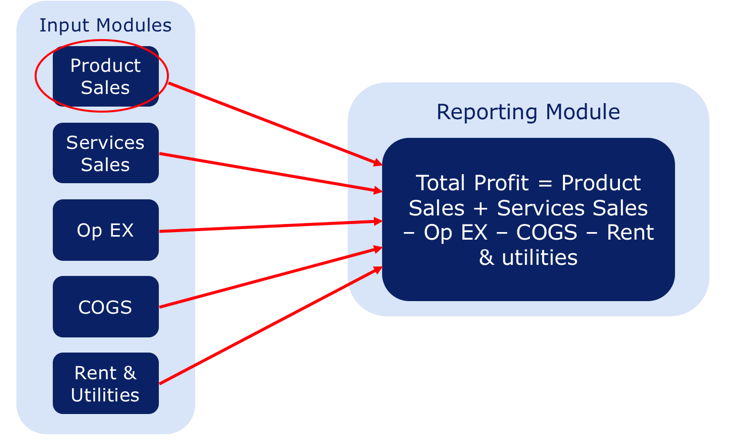What happens when one of the components of COGS changes? Since all the source components are included in the formula, when anything within any of the components changes, this formula is recalculated. If there are a significant number of component expressions, this can put a larger overhead on the calculation engine than is necessary.

There is a simple way to structure the module to lessen the demand on the calculation engine. You can separate the input lines in the reporting module by creating a line item for each of the components and adding the Total Profit formula as a separate line item. This way, changes to the source data only cause the relevant line item to recalculate.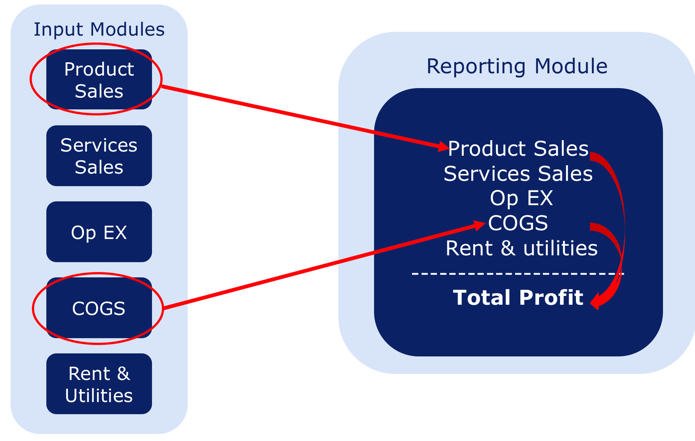For example, a change in the Product Sales calculation only affects the Product Sales and the Total Profit line items in the Reporting module; Services Sales, Op EX, COGS and Rent & Utilities are unchanged. Similarly, a change in COGS only affects COGS and Total Profit in the Reporting module.

Keep the general guidelines in mind. It is not practical to have every downstream formula broken out into individual line items.

## Plan to provide early exits from formulas

Conditional formulas (IF/THEN) present a challenge for the model builder in terms of what is the optimal construction for the formula, without making it overly complicated and difficult to read or understand. The basic principle is to avoid making the calculation engine do more work than necessary. Try to set up the formula to finish the calculations as soon as possible.

Always put first the condition that is most likely to occur. That way the calculation engine can quit the processing of the expression at the earliest opportunity.

Here is an example that evaluates Seasonal Marketing Promotions: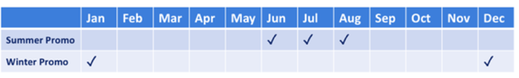The summer promotion runs for three months and the winter promotion for two months.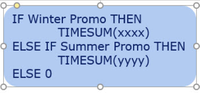There are more months when there is no promotion, so this formula is not optimal and will take longer to calculate.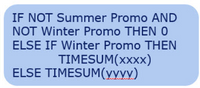This is better, as the formula will exit after the first condition more frequently.

There is an even better way to do this. Following the principles from above, add another line item for no promotion.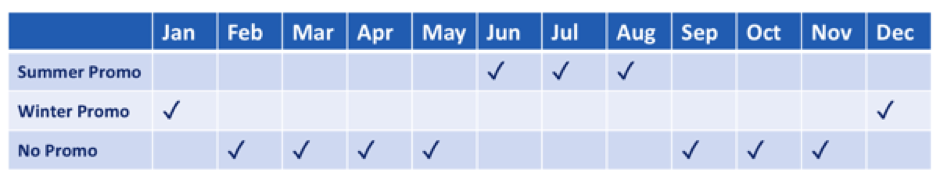And then the formula can become: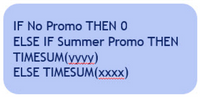This is even better because the calculation for No Promo has already been calculated, and Summer Promo occurs more frequently than Winter Promo.

It is not always clear which condition will occur more frequently than others, but here are a few more examples of how to optimize formulas:

• FINDITEM formula
The Finditem element of a formula will work its way through the whole list looking for the text item, and if it does not find the referenced text, it will return blank. If the referenced text is blank, it will also return a blank. Inserting a conditional expression at the beginning of the formula keeps the calculation engine from being overtaxed.
1. IF ISNOTBLANK(TEXT) THEN FINDITEM(LIST,TEXT) ELSE BLANK
2. Or
3. IF BLANK(TEXT) THEN BLANK ELSE FINDITEM(LIST,TEXT)
Use the first expression if most of the referenced text contains data and the second expression if there are more blanks than data.
• LAG, OFFSET, POST, etc.
If in some situations there is no need to lag or offset data, for example, if the lag or offset parameter is 0. The value of the calculation is the same as the period in question. Adding a conditional at the beginning of the formula will help eliminate unnecessary calculations:
1. IF lag_parameter = 0 THEN 0 ELSE LAG(Lineitem, lag_parameter, 0)
2. Or
3. IF lag_parameter <> 0 THEN LAG(Lineitem, lag_parameter, 0) ELSE 0
The use of formula a or b will depend on the most likely occurrence of 0s in the lag parameter.
• Booleans
Avoid adding unnecessary clutter for line items formatted as BOOLEANS. There is no need to include the TRUE or FALSE expression, as the condition will evaluate to TRUE or FALSE.
1. Sales>0
3. IF Sales > 0 then TRUE ELSE FALSE

Author David Smith.

Tagged:

•Great recommendations!

•Hi David,

I have a question regarding FINDITEM tip. For example we know that we'll have max 5% text fields as a BLANK. With your proposal we save FINDITEM lookup time for those cases only (which is 5%) and we make engine to compute IF statements for 95% of other cells. Doesn't it make calculation slower in such cases?

Thanks,

Hayk

•Hi Hayk

The check for a blank is very fast, but wether this outweighs the time to traverse the list is more difficult to prove.  If the list is very large (say over 1 million items), I think it would be worth the check.  For smaller lists with a small percentage of blank, it is probably not needed.

As with a lot of modelling decisions, the answer is often "it depends", we tried to propose a Best Practice that would cover most situations and provide a solution or way of thinking if you noticed some performance degredation.  We have seen some huge performance gains from putting in the checks.

What I would say, it is always best to check.  If you remove the formula, hit enter and then paste it again and hit enter, this will force a full recalculation of the line item.  So, time it with the check and then time it without.

Let me know what you find out, but we are going to run some internal tests to see if there is a threshold after which it makes a significant difference.

It is an interesting question and there are some functions that have a built in check for blanks, but FINDITEM isn't one of then; we have asked if we can include the built in check, which will negate the need for this checking.

Thank you again, because this kind of conversation prompts discussion and makes the product better.

•Hi David,

Thank you for explanation, it is always good to know some background.

I've tested what you proposed on a flat-list module with 14K cells (1400 of them are blank). Also created a module with 200mln cells based on that flat-list. And can't see the difference in both cases. Guess it should be measured with some tools.

Thanks,
Hayk

•These recommendations are great, but far too often formulas are not this simple to break up or  reeningeer to optimize in such a clean manner.  When formulas get really long and complex, it is difficult to know where and how to break it up into manageable pieces.  Is it possible to get more complex examples than the ones above and provide some guidance on best practices on how deal with them? I have seen one at my clients that is nearly three pages long in a word document and trying to figure a way to break it apart makes my head spin.

•with Finditem is there a difference between

IF ISNOTBLANK(TEXT) THEN FINDITEM(LIST,TEXT) ELSE BLANK

VS

IF ISNOTBLANK(FINDITEM(LIST,TEXT)) THEN FINDITEM(LIST,TEXT) ELSE BLANK

in-terms of performance ?

•It is always better in terms of performance to split components out so the first one will be more efficient.

Also, you can see that the expression FINDITEM(list, text) is repeated

"calc once, reference many times"

Also you could even get rid of the ISNOTBLANK if you know there are no blanks in the data

FINDITEM(Item, Text) is fine in that case

Hope that helps

David

•Instead of IF else, can we use Factor or Multiplier,

For eg. We have the formula IF Weekly_Factor=1 then Weekly_Revenue else Monthly_Revenue

can we re-structure it to Weekly_Revenue*Weekly_Factor+Monthly_Revenue*Monthly_Factor

will it help in improving Performance